# GSEB Solutions Class 12 Maths Chapter 9 Differential Equations Ex 9.5

Gujarat Board GSEB Textbook Solutions Class 12 Maths Chapter 9 Differential Equations Ex 9.5 Textbook Questions and Answers.

## Gujarat Board Textbook Solutions Class 12 Maths Chapter 9 Differential Equations Ex 9.5

In each of the questions 1 to 10, show that the given differential equation is homogeneous and solve each of them:
Question 1.
(x2 + x dy) = (x2 + y2) dx
Solution:
(x2 + xy) dy = (x2 + y2)dx
or $$\frac{dy}{dx}$$ = $$\frac{x^{2}+y^{2}}{x^{2}+x y}$$ = f(x, y) (say) …………….. (1)
where f(x, y) = $$\frac{x^{2}+y^{2}}{x^{2}+x y}$$
Replacing by λx and y by λy, we getHence, f(x, y) is a homogeneous function of degree zer0.
To solve it, put y = vx or
$$\frac{dy}{dx}$$ = v + x $$\frac{dv}{dx}$$
Equation (1) may be written as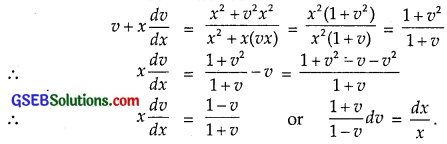Integrating, we getPut v = $$\frac{y}{x}$$, we get∴ Solution is (x – y)2 = Cxe-y/x.Question 2.
y’ = $$\frac{x+y}{x}$$
Solution:
y’ = $$\frac{x+y}{x}$$
∴ $$\frac{dy}{dx}$$ = f(x, y), where f(x, y) = $$\frac{x+y}{x}$$.
Replacing x by λx and y by λy,∴ f(x, y) is a homogeneous function of degree zero.Putting v = $$\frac{y}{x}$$, we get $$\frac{y}{x}$$ = log |x| + C or y = x log |x| + Cx.

Question 3.
(x – y)dy – (x + y)dx = 0
Solution:
(x – y) dy – (x + y)dx = 0
or $$\frac{dy}{dx}$$ = $$\frac{x+y}{x-y}$$ = f(x, y)
∴ f(x, y) = $$\frac{x+y}{x-y}$$
Replacing x by λx and y by λy,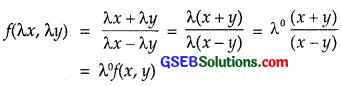⇒ f(x, y) is a homogeneous function of degree zero.
Put y = vx so thatIntegrating, we getQuestion 4.
(x2 – y2)dx + 2xy dy = 0
Solution: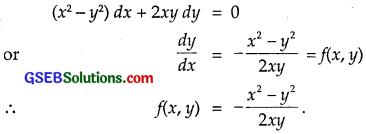Replacing x by λx and y by λy, we getHence, f(x, y) is a homogeneous functoin of degree zero.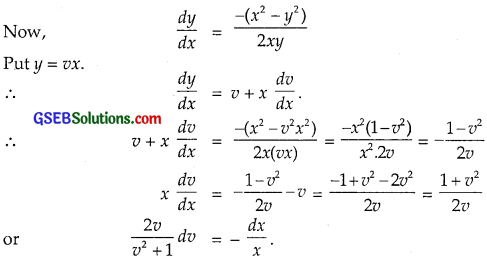Integrating, we get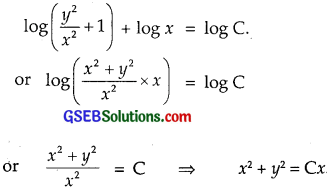Question 5.
x2 $$\frac{dy}{dx}$$ = x2 – 2y2 + xy
Solution: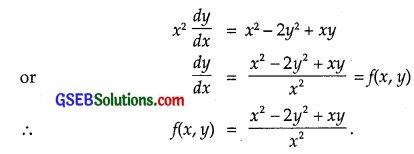Replacing x by λx and y by λy in f(x, y), we get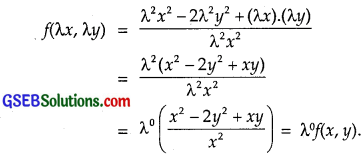∴ f(x, y) is a homogenous function of degree zero.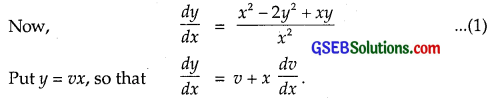Putting these values in (1), we get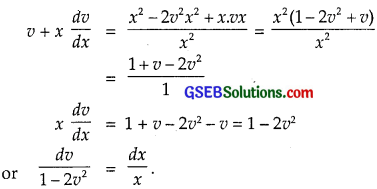Integrating, we have:Put $$\sqrt{2}$$v = t so that $$\sqrt{2}$$dv = dt.Put v = $$\frac{y}{x}$$, we get the solution as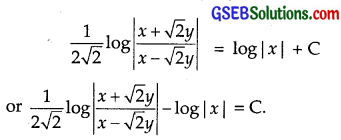Question 6.
x dy – y dx = $$\sqrt{x^{2}+y^{2}}$$ dx
Solution:Replacing x by λx and y by λy in f(x, y), we get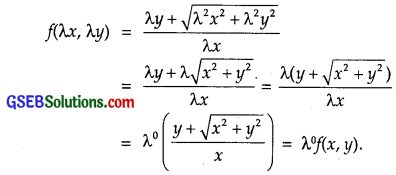∴ f(x, y) is a homogenous function of degree zero.Putting these values in (1), we getIntegrating, we have: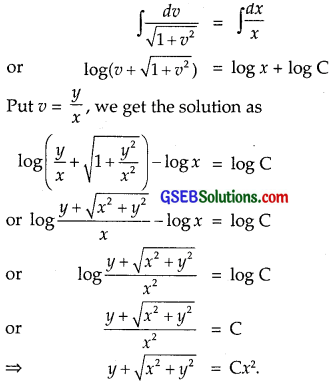Question 7.
{x cos ($$\frac{y}{x}$$) + y sin ($$\frac{y}{x}$$)} y dx = {y sin ($$\frac{y}{x}$$) – x cos ($$\frac{y}{x}$$)} x dy
Solution: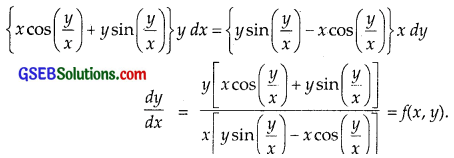Replacing x by λx and y by λy in f(x, y), we get∴ f(x, y) is a homogenous function of degree zero.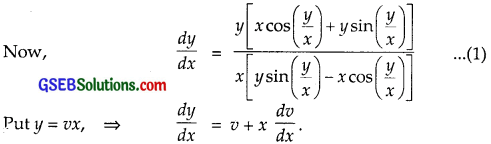Putting in these values in (1), we get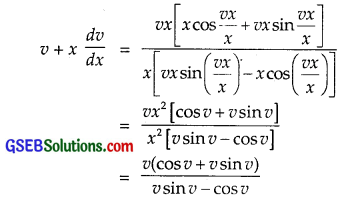Transposing v to R.H.S., we getIntegrating, we getQuestion 8.
x $$\frac{dy}{dx}$$ – y + x sin $$\frac{y}{x}$$ = 0
Solution: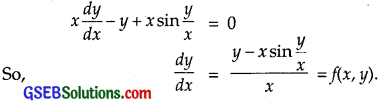Replacing x by λx and y by λy in f(x, y), we get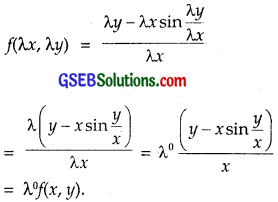∴ f(x, y) is a homogenous function of degree zero.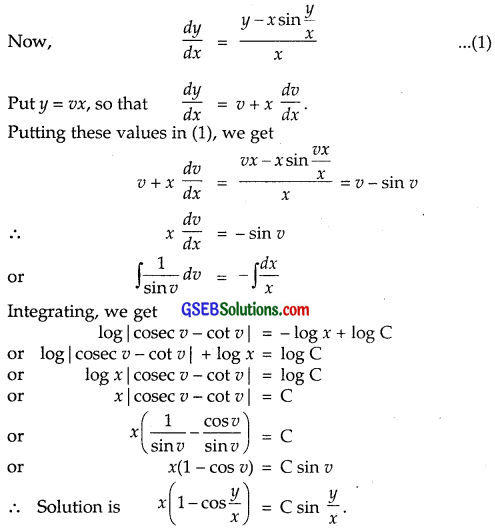Question 9.
y dx + xlog ($$\frac{y}{x}$$)dy – 2x dy = 0
Solution: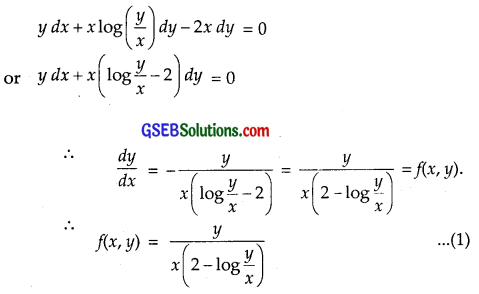Replacing x by λx and y by λy in f(x, y), we getPut y = vx, so that
$$\frac{dy}{dx}$$ = v + x $$\frac{dv}{dx}$$.
Putting these values in (2), we getIntegrating, we getPut log v – 1 = t, so that $$\frac{1}{v}$$dv = dt
Therefore, from (3), we get
– log v + ∫ $$\frac{dt}{t}$$ = log x + log C
⇒ – log v + log t = log x + log C
or – log v + log (log v – 1) = log x + log C
or log (log v – 1) = log v + log x + log C
= log v Cx
Put v = $$\frac{y}{x}$$. Then the solution is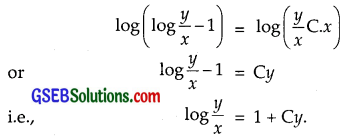Question 10.
(1 + ex/y)dx + ex/y(1 – $$\frac{x}{y}$$)dy = 0
Solution:Replacing x by λx and y by λy and obtain:Hence, f(x, y) is a homogeneous function of degree zero.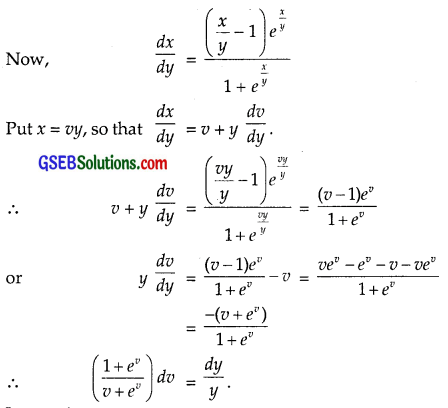Integrating, we get∴ Required solution is x + y ex/y = Cy.

For each of the differential equations in questions 11 to 15, find the particular solution satisfying the given condition:

Question 11.
(x + y)dy + (x – y)dx = 0, y = 1, when x = 1.
Solution:
(x + y)dy + (x – y)dx
or (x + y) dy = – (x – y) dx
∴ $$\frac{dy}{dx}$$ = – $$\frac{x-y}{x+y}$$ = f(x, y).
f(x, y) is a homogeneous function.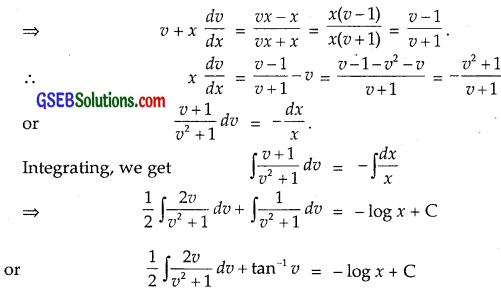Put v2 + 1 = t so that 2v dv = dt
or $$\frac{1}{2}$$ ∫$$\frac{dt}{t}$$ + tan-1 v = – log x + C
or $$\frac{1}{2}$$log t + tan-1v = – log x + C
or $$\frac{1}{2}$$log(v2 + 1) + tan-1 v = – log x + C
Put v = $$\frac{y}{x}$$ and obtain:Put x = 1, y = 1 and obtain:
$$\frac{1}{2}$$log(1 + 1) + tan-1$$\frac{1}{1}$$ = C
or $$\frac{1}{2}$$log 2 + $$\frac{π}{4}$$ = C
Putting value of C in (1). The particular solution is
$$\frac{1}{2}$$log(x2 + y2) + tan-1($$\frac{y}{x}$$)
= $$\frac{1}{2}$$ log 2 + $$\frac{π}{4}$$.Question 12.
x2dy + (xy + y2)dx = 0, y = 1, when x = 1.
Solution:
x2dy + (xy + y2) dx = 0
∴ $$\frac{dy}{dx}$$ = $$\frac{x y+y^{2}}{x^{2}}$$ = f(x, y)
f(x, y) is homogeneous
∴ Put y = vx, so that $$\frac{dy}{dx}$$ = v + x $$\frac{dv}{dx}$$.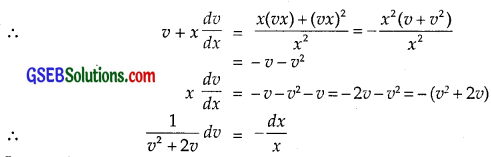Integrating, we get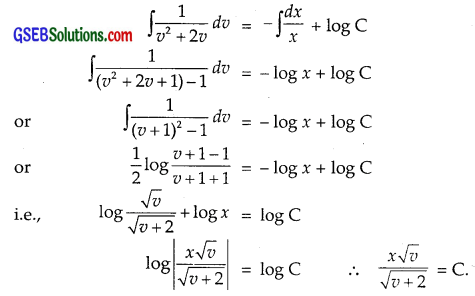Putting v = $$\frac{y}{x}$$, we get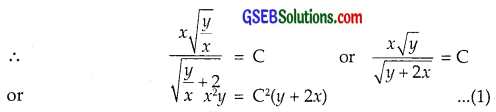Putting x = 1, y = 1, we get
1 = C2(1 + 2) ∴ C2 = $$\frac{1}{3}$$.
Putting C2 = $$\frac{1}{3}$$ in (1), we get
x2y = $$\frac{1}{3}$$(y + 2x)
∴ Particular solution is 3x2y = y + 2x.

Question 13.
(x sin2 $$\frac{y}{x}$$ – y) dx + x dy = 0, y = $$\frac{π}{4}$$, when x = 1.
Solution:
[x sin2 $$\frac{y}{x}$$ – y] dx + x dy = 0which is homogeneous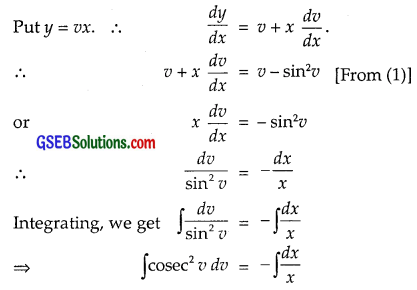⇒ – cot v = – log x + C
⇒ + log x – cot v = C
Putting v = $$\frac{y}{x}$$, we get the general solution as
log x – cot $$\frac{y}{x}$$ = C.
Putting x = 1 and y = $$\frac{π}{4}$$, we get
log 1 – cot $$\frac{π}{4}$$ = C or 0 – 1 = C ⇒ C = – 1.
∴ Particular solution is
log x – cot $$\frac{y}{x}$$ = – 1 or cot $$\frac{y}{x}$$ – log x = 1.Question 14.
$$\frac{dy}{dx}$$ – $$\frac{y}{x}$$ + cosec ($$\frac{y}{x}$$) = 0, y = 0, when x = 1.
Solution:
We have:
$$\frac{dy}{dx}$$ – $$\frac{y}{x}$$ + cosec $$\frac{y}{x}$$ = 0 ……………. (1)
Put y = vx, so that $$\frac{dy}{dx}$$ = v + x $$\frac{dv}{dx}$$. ………………….. (2)
From (1) and (2), we get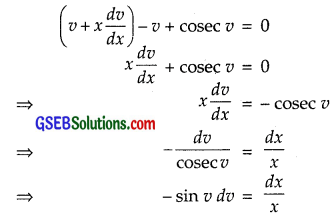Integrating both sides, we get
∫(- sin v)dv = ∫$$\frac{dx}{x}$$
⇒ cos v = log |x| + C
⇒ cos $$\frac{y}{x}$$ = log|x| + C
It is given that y(1) = 0, i.e; when x = 1, y = 0.
∴ cos 0 = log|1| + C
⇒ 1 = 0 + C ⇒ C = 1.
∴ cos $$\frac{y}{x}$$ = log |x| + 1
⇒ log |x| = cos $$\frac{y}{x}$$ – 1, (x ≠ 0)
which is the required particular solution.Question 15.
2 xy + y2 – 2x2 $$\frac{dy}{dx}$$ = 0, y = 2, when x = 1.
Solution:
We have: 2xy + y2 – 2x2 $$\frac{dy}{dx}$$ = 0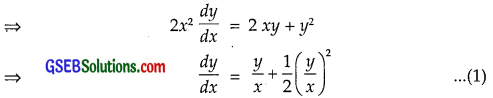which is a homogeneous differential equation.
Put y = vx. Then $$\frac{dy}{dx}$$ = v + x $$\frac{dv}{dx}$$.
∴ (1) becomes v + x $$\frac{dv}{dx}$$ = v + $$\frac{1}{2}$$v2
⇒ x $$\frac{dv}{dx}$$ = $$\frac{1}{2}$$ v2 ⇒ $$\frac{2}{v^{2}}$$dv = $$\frac{dx}{x}$$.
Integrating both sides, we get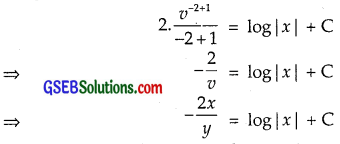It is given that y(1) = 2, i.e., when x = 1, y = 2.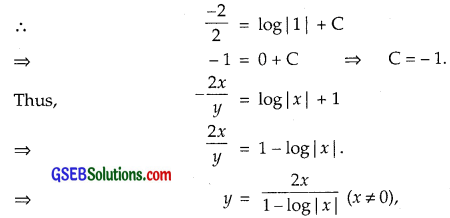which is the required particular solution.

Choose the correct answers in the following questions 16 and 17:

Question 16.
A homogeneous differential equation of the form $$\frac{dx}{dy}$$ = h($$\frac{x}{y}$$) can be solved by making the substitution.
(A) y = vx
(B) v = yx
(C) x = vy
(D) x = v
Solution:
For solving the homogeneous equation of the form $$\frac{dx}{dy}$$ = h($$\frac{x}{y}$$), we make the substitution x = vy.
∴ Part (C) is the correct answer.Question 17.
Which of the following is a homogeneous differential equation?
(A) (4x + 6y + 5)dy – (3y + 2x + 4) dx = 0
(B) xy dx – (x3 + y3)dy = 0
(C) (x3 + 2y2) dx + 2xy dy = 0
(D) y2dx + (x2 – xy – y2) dy = 0
Solution:
Consider the differential equation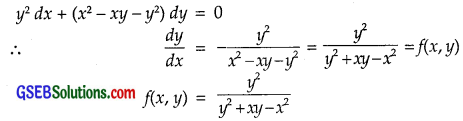Replacing x by λx by λy, we get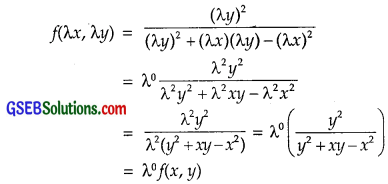∴ f(x, y) is the homogeneous function of degree zero.
Hence, part (D) is the correct answer.